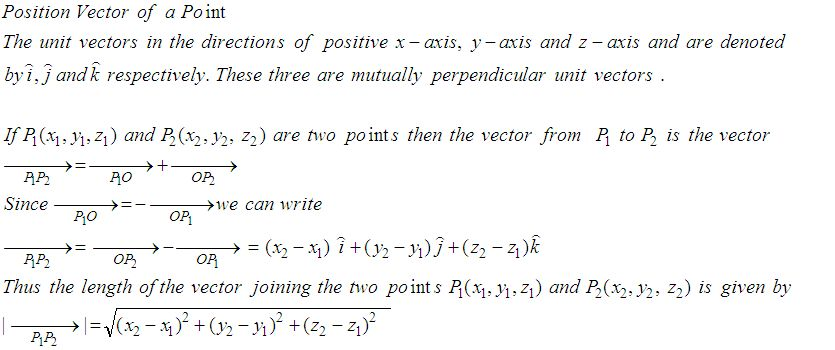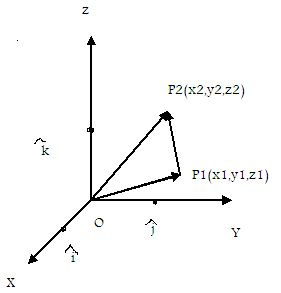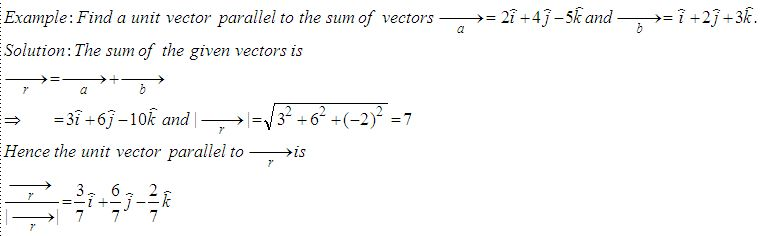Name: ___________________Date:___________________

 Email us to get an instant 20% discount on highly effective K-12 Math & English kwizNET Programs!

High School Mathematics - 25.9 Position Vector of a PointDirections: Answer the following.
 Q 1: Find the vector joining the initial point P(-4,2) and terminal point Q(0,-4) 8i-6j4i-6j4i+6j Q 2: Find a unit vector in the direction from P(3,2) towards Q(5,6).4i+52i+3i/Ö5 + 2j/Ö5 Q 3: Find a unit vector parallel to the sum of vectors a = 2i+4j-5k, b = i+2j+3k8i+4j-3k3/7i + 6/7j - 2/7 k2-i8 Q 4: If P = 2,4,7 and P2 = (-4, -1, 5) then find vector P1P2.6i+5j+3k-6i+5j-6i-5j-2k Q 5: If P = 2,4,7 and P2 = (-4, -1, 5) then find the magnitude of vector P1P2.Ö656i+5j3i+6j Q 6: Find the vector with initial point P(6,-2) and terminal point Q(4,-8).-2i+10j8i+2j6i-9j Q 7: Find the vector in the direction of the vector -i+2j+2k that has magnitude 7.-3i+2j7(-i+2j+2k)/3i-j+k Q 8: Find the unit vector in the direction of P(1,2,3) towards Q(4,5,6).(i+j+k)/Ö36i+5j+2k8j-2k Question 9: This question is available to subscribers only! Question 10: This question is available to subscribers only!